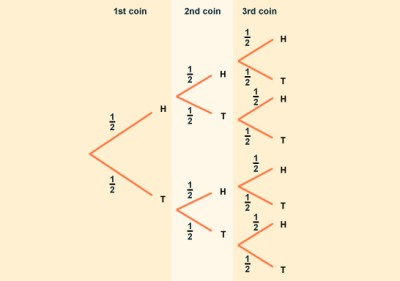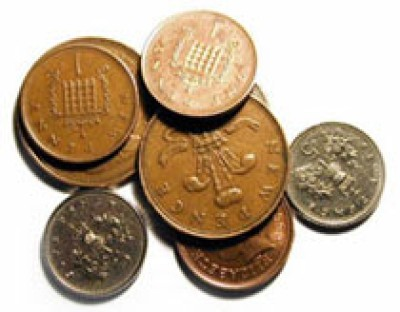List of public pages created with Protopage

# Home

## Plain sticky notes

### Hello - Bonjour - Hola - Cuao

welcome to my proto page! its so fun and mathmatical!

## Todo lists

### Todo listMaths Unit AReviseRevise

# Factorising

## Plain sticky notes

### Intro

You can also factorise quadratic expressions. Remember that factorising an expression simplifies it in some way. Factorising is the reverse of expanding brackets. Factorising quadratic expressions ( x + 2 ) ( x + 5 ) = x2 + 7x + 10 ( x + 2 ) ( x + 3 ) = x2 + 5x + 6 ( x - 3 ) ( x - 5 ) = x 2 - 8x + 15 ( x + 6 ) ( x - 5 ) = x2 + x - 30 ( x - 6 ) ( x + 5 ) = x2 - x - 30
Factorising: To factorise an expression such x2 + 5x + 6, you need to look for two numbers that add up to make 5 and multiply to give 6. The factor pairs of 6 are: 1 and 6 2 and 3 2 and 3 add up to 5. So: (x +2) (x+3) = x2 + 5x + 6 ~Factorising expressions gets trickier with negative numbers.

# Probability

## Plain sticky notes

### Sticky note

If you are studying the higher paper, you will also need to know about tree diagrams, the and/or rule and conditional probabilities. Tree diagrams Tree diagrams allow us to see all the possible outcomes of an event and calculate their probability. Each branch in a tree diagram represents a possible outcome. If two events are independent, the outcome of one has no effect on the outcome of the other. For example, if we toss two coins, getting heads with the first coin will not affect the probability of getting heads with the second. From the tree diagram, we can see that there are eight possible outcomes. To find out the probability of a particular outcome, we need to look at all the available paths (set of branches). The sum of the probabilities for any set of branches is always 1. Also note that in a tree diagram to find a probability of an outcome we multiply along the branches and add vertically. The probability of three heads is: P (H H H) = 1/2 × 1/2 × 1/2 = 1/8 P (2 Heads and a Tail) = P (H H T) + P (H T H) + P (T H H) = 1/2 × 1/2 × 1/2 + 1/2 × 1/2 × 1/2 + 1/2 × 1/2 × 1/2 = 1/8 + 1/8 + 1/8 = 3/8

## Photos

### This is a tree diagram:# Bookmarks

## Plain sticky notes

### Sticky note

Click 'edit' on the Bookmarks widget to add all of your favorite sites to your page for quick access